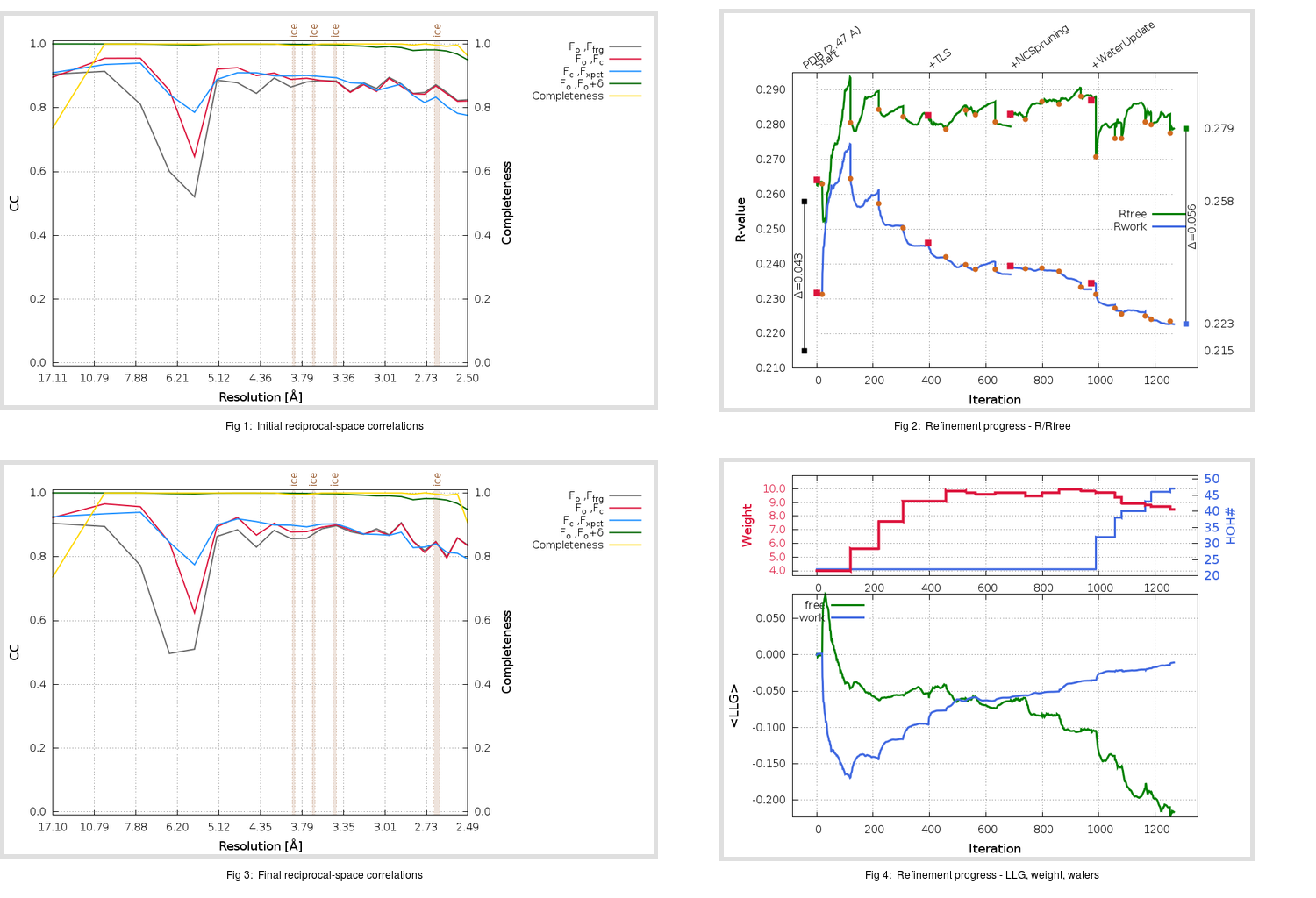Content:

```    Diffraction limits & principal axes of ellipsoid fitted to diffraction cut-off surface:
2.333         1.0000   0.0000   0.0000       a*
2.382         0.0000   1.0000   0.0000       b*
2.429         0.0000   0.0000   1.0000       c*
```

## Deposited

` `
 Date deposited Date data collection Resolution R, Rfree 20200204 20200204 2.47 0.2150 0.2580

Molprobity (CCP4 7.0 version) summary:

```Ramachandran outliers =   0.00 %
favored =  99.22 %
Rotamer outliers      =   0.00 %
C-beta deviations     =     0
Clashscore            =   3.75
RMS(bonds)            =   0.0059
RMS(angles)           =   1.03
MolProbity score      =   1.16
Resolution            =   2.47
R-work                =   0.2150
R-free                =   0.2580
```

```Number of waters      =    22

<B> (all atoms) =   38.51 ( sd =   14.58 ) for       1078 non-hydrogen atoms
<B>   (protein) =   38.48 ( sd =   14.69 ) for       1056 non-hydrogen atoms
<B>     (water) =   40.20 ( sd =    7.55 ) for         22 non-hydrogen atoms
<B>    (others) =    0.00 ( sd =    0.00 ) for          0 non-hydrogen atoms

B min/max       (all non-hydrogen atoms) =   17.49 /   99.12
B min/max   (protein non-hydrogen atoms) =   17.49 /   99.12
B min/max     (water non-hydrogen atoms) =   23.74 /   51.92
B min/max     (other non-hydrogen atoms) =    0.00 /    0.00
```

## BUSTER (re-)refinement

` `

Molprobity (CCP4 7.0 version) summary:

```Ramachandran outliers =   0.00 %
favored = 100.00 %
Rotamer outliers      =   6.45 %
C-beta deviations     =     0
Clashscore            =   1.40
RMS(bonds)            =   0.0116
RMS(angles)           =   1.26
MolProbity score      =   1.49
Resolution            =   2.47
R-work                =   0.2225
R-free                =   0.2791
```

```Number of waters      =    47

<B> (all atoms) =   39.05 ( sd =   10.76 ) for       1103 non-hydrogen atoms
<B>   (protein) =   38.95 ( sd =   10.81 ) for       1056 non-hydrogen atoms
<B>     (water) =   41.37 ( sd =    9.02 ) for         47 non-hydrogen atoms
<B>    (others) =    0.00 ( sd =    0.00 ) for          0 non-hydrogen atoms

B min/max       (all non-hydrogen atoms) =   21.88 /   81.41
B min/max   (protein non-hydrogen atoms) =   21.88 /   81.41
B min/max     (water non-hydrogen atoms) =   26.28 /   63.03
B min/max     (other non-hydrogen atoms) =    0.00 /    0.00
```

Refinement progression:Results:

` `
 File Remark 6LVN_aB_refine.01_04_refine.pdb.gz exact refinement commands are in header 6LVN_aB_refine.01_04_refine.mtz.gz including original deposited data and several re-refinement map coefficients 6LVN_aB_refine.01_04_BUSTER_model.cif.gz including any non-standard compound restraints 6LVN_aB_refine.01_04_BUSTER_refln.cif.gz